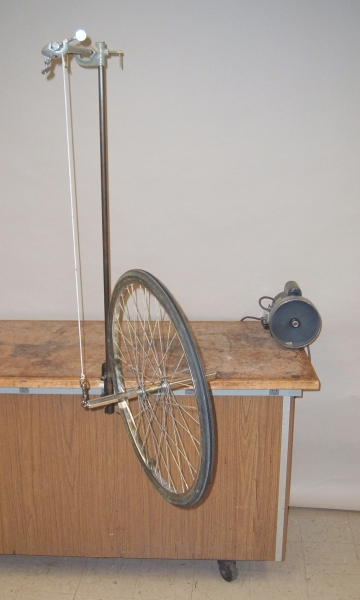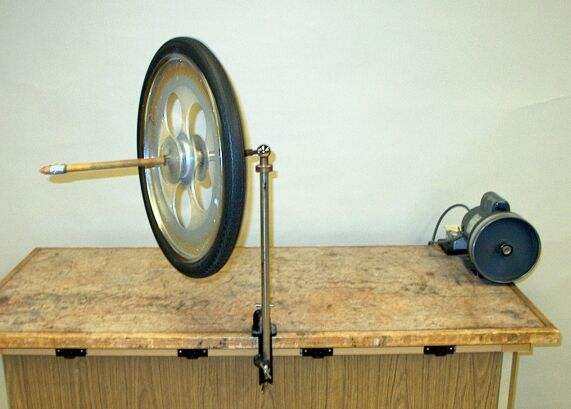With either of these two wheels and mounts, you can demonstrate precession of a spinning bicycle wheel. Switch on the motor, and holding the axle horizontal in front of you, press the tire firmly against the large pulley to get the wheel spinning. For the wheel at left, attach the eye at one end of the axle to the snap hook and release the wheel. You can  then suspend the wheel from the eye at the other end of the axle to show that this reverses the direction of precession. For the wheel at right, set the ball end of the axle in the cup at the top of the post and release the wheel. (The motor is usually mounted on the main demonstration table.) A short rope with a wooden handle on one end and a swivel snap hook on the other, is available for using the hanging bicycle wheel at a remote location. It is possible to spin the wheel fast enough by hand that doing this and then suspending it by the rope with the handle provides a useful demonstration.

The physics illustrated in this demonstration is similar or identical to that in demonstrations 28.54 -- Bicycle wheel as a top, 28.60 -- Maxwell’s top, and 28.63 -- Rotating wheel in suitcase.

Just as an object moving in a straight line has linear momentum, a rotating object has angular momentum. This angular momentum is L = Iω, where I is the moment of inertia of the object, and ω is its angular velocity. L points along the axis about which the object is rotating, and its sense is given by the right-hand rule. If you curl the fingers of your right hand in the direction in which the object is rotating, your extended thumb points in the direction of L. The motor pulley as it is shown in the photograph above rotates in the clockwise direction. So when you hold one of the bicycle wheels against it, it spins the bicycle wheel counterclockwise. Its angular momentum vector L, then, points toward the front of the table, or to your left.

If the large bicycle wheel is not spinning, and you hang it from the eye at either end of the axle, gravity exerts a torque on the wheel at its center of mass, about the suspension point, and the wheel merely falls; the plane of the wheel ends up horizontal, and the axle is vertical. If you set the wheel spinning as described above, however, and now hang it from the eye at the left end of the axle, gravity still exerts this torque, but the wheel now has angular momentum and it cannot fall, but must move in the direction dictated by the change in angular momentum introduced by the torque exerted by gravity; it precesses. As we will see below, when you hang the spinning wheel from the left end of its axle, then, instead of falling, it rotates about the suspension point in a clockwise direction as viewed from above. A snapshot of this is shown at left in the photograph above.

Similarly, if the small bicycle wheel is not spinning, and you set it on the ball end of its axle, gravity exerts a torque on the wheel at its center of mass, about the ball end, and the wheel merely falls. (Do not do this with the small wheel. If it falls off the table, or worse, the pivot cup, the axle will be severely bent upon the wheel’s impact with the floor.) If, however, you set the wheel spinning, and now set the ball end in the pivot cup, gravity still exerts this torque, but the wheel now has angular momentum and it cannot fall, but must move in the direction dictated by the change in angular momentum introduced by the torque exerted by gravity; it precesses. A snapshot of this is shown at right in the photograph above.

If r is the position of the center of mass relative to the suspension point for the hanging wheel, or the ball end for the small wheel, the torque from gravity is τ = r × mg, where m is the mass of the wheel and g is the acceleration due to gravity. It points outward from the suspension or pivot point, along the horizontal plane through that point, perpendular to r and mg, and rotates about the pivot point as the wheel precesses. The change in angular momentum equals this torque, or τ = dL/dt. Thus, the change in angular momentum – the precession – is in the direction of the torque exerted by gravity, and for a small time interval, ΔL = τΔt. L + ΔL has the same magnitude as L, but its head is displaced with respect to that of L by an angle φ, the angle made by the (horizontal) radii from L and L + ΔL to the axis through the pivot point. (If we place the pivot point at the origin of a set of coordinate axes, this is the z- axis. If L and L + ΔL are also horizontal, these radii lie along them.) The rate at which L precesses, then, is ωp = Δφt. If we call the angle that L makes with the pivot axis, θ, then the radius from the tip of L to the pivot axis is L sin θ. (θ is also the angle that mg makes with r.) Since ΔL is very small compared to L (and to L sin θ), for an incremental change in φ, Δφ ≅ ΔL/L sin θ, and Δφ = τ Δt/L sin θ, so ωp = τ/L sin θ. τ = rmg sin θ, so ωp = rmg/L, or ωp = rmg/. (If we rewrite ωp = τ/L sin θ as τ = ωp L sin θ, we see that this torque is also the cross product of the precession velocity and the angular momentum: τ = ωp × L.)

Thus, if you set the large wheel spinning and hang it from the eye at the left end of the axle, L points in toward the suspension point, and the wheel precesses in the clockwise direction as viewed from the top. (Imagine moving the tip of L in the direction of τ.) You can also hang the wheel from the eye at the right end of the axle. If you do this, L now points outward from the suspension point, and the wheel now precesses in the counterclockwise direction as viewed from above.

As mentioned and shown above, the smaller wheel has a ball at one end of its axle, which you can set on the pivot at the top of the rod mounted to the table. If you hold the wheel with the ball end to your left, when you set it spinning with the motor, its angular momentum points to your left, toward the ball. When you set the ball on the pivot, L points toward the pivot, and the wheel precesses in the clockwise direction as viewed from the top. If you set the wheel spinning with the ball end on your right, its angular momentum now points away from the ball end. When you set the ball on the pivot, L points away from the pivot, and the wheel precesses in the counterclockwise direction as viewed from above.

References:

1) Resnick, Robert and Halliday, David. Physics, Part One, Third Edition (New York: John Wiley and Sons, 1977), pp. 260-262.
2) http://hyperphysics.phy-astr.gsu.edu entry on precession of a spinning top.
3) http://hyperphysics.phy-astr.gsu.edu/hbase/rotv2.html#rvec4 entry on precession torque.